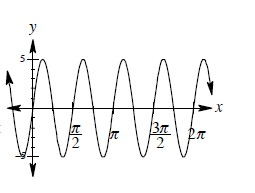### Home > AC > Chapter 16 > Lesson 16.9.1.2 > Problem9-26

9-26.

Consider the graph of $y = \sin x$. How would you change the equation to obtain a graph that looks like the one below?

1. Change the amplitude to $5$.
2. There are now $4$ cycles in $2π$ instead of $1$.
So change the frequency '$b$'.